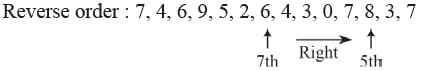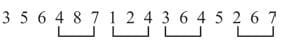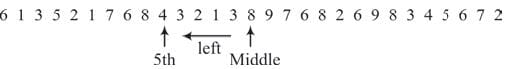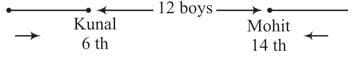Olympiad Test: Number Ranking - 2

# Olympiad Test: Number Ranking - 2 - Class 8

Test Description

## 15 Questions MCQ Test Mathematical Olympiad Class 8 - Olympiad Test: Number Ranking - 2

Olympiad Test: Number Ranking - 2 for Class 8 2023 is part of Mathematical Olympiad Class 8 preparation. The Olympiad Test: Number Ranking - 2 questions and answers have been prepared according to the Class 8 exam syllabus.The Olympiad Test: Number Ranking - 2 MCQs are made for Class 8 2023 Exam. Find important definitions, questions, notes, meanings, examples, exercises, MCQs and online tests for Olympiad Test: Number Ranking - 2 below.
 1 Crore+ students have signed up on EduRev. Have you?
Olympiad Test: Number Ranking - 2 - Question 1

### What is the difference between the sum of even digits and sum of odd digits in the number 7 4 6 2 5 8 9 3?

Detailed Solution for Olympiad Test: Number Ranking - 2 - Question 1

Sum of even digits = 4 + 6 + 2 + 8 =20
Sum of odd digits = 7 + 5 + 9 + 3 = 24
Difference = 24 – 20 = 4.

Olympiad Test: Number Ranking - 2 - Question 2

### If the given series is written in the reverse order which number will be 5th to the right of the seventh number from the left? 7, 3, 8, 7, 0, 3, 4, 6, 2, 5, 9, 6, 4, 7.

Detailed Solution for Olympiad Test: Number Ranking - 2 - Question 2

Given series 7, 3, 8, 7, 0, 3, 4, 6, 2, 5 , 9, 6, 4, 7Olympiad Test: Number Ranking - 2 - Question 3

### In the given number sequence, how many such even numbers which are exactly divisible by its immediate preceding number but not exactly divisible by its immediate succeeding number? 3 5 6 4 8 7 1 2 4 3 6 4 5 2 6 7

Detailed Solution for Olympiad Test: Number Ranking - 2 - Question 3Olympiad Test: Number Ranking - 2 - Question 4

From the given numbers
823, 716, 539, 683, 937
what will be the first digit of the second highest number after the position of only second and third digits within each number are interchanged?

Detailed Solution for Olympiad Test: Number Ranking - 2 - Question 4

Given numbers – 823, 716, 539, 683, 937
New numbers – 832, 761, 593, 638, 973
Second highest number = 832.
Ist first digit = 8

Olympiad Test: Number Ranking - 2 - Question 5

From the given numbers
728, 593, 645, 296, 968
what will be the first digit of the second highest number after the position of only first and third digits within each number are interchanged?

Detailed Solution for Olympiad Test: Number Ranking - 2 - Question 5

Given numbers – 728, 593, 645, 296, 968
New numbers – 827, 395, 546, 692, 869
Second highest number = 827
Ist first digit = 8

Olympiad Test: Number Ranking - 2 - Question 6

Rohit is 14th from the right end in a row of 70 students. What is his position from left end?

Detailed Solution for Olympiad Test: Number Ranking - 2 - Question 6

Numbers of students towards left to Rohit = Rohit’s position from left = 56 + 1 = 57th

Olympiad Test: Number Ranking - 2 - Question 7

Manak ranks 5th from the top and thirdseventh from the bottom in a class, How many students are there in the class?

Detailed Solution for Olympiad Test: Number Ranking - 2 - Question 7

Total number of students = 4 + 1 + 36 = 41.

Olympiad Test: Number Ranking - 2 - Question 8

Which is the 5th number to the left of the number which is exactly in the middle of the given sequence of numbers?
6 1 3 5 21 7 6 8 4 3 2 1 3 8 9 7 6 8 2 6 9 8 3 4 5 6 7 2

Detailed Solution for Olympiad Test: Number Ranking - 2 - Question 8Total numbers = 29.
Middle number = 15th number

Olympiad Test: Number Ranking - 2 - Question 9

How many numbers from 50 to 150 are there each of which is exactly divisible by 4 and also has 4 as a digit?

Detailed Solution for Olympiad Test: Number Ranking - 2 - Question 9

The numbers divisible by 4 from 50 to 150 are 52, 56, 60, 64, 68, 72, 76, 80, 84, 88, 92, 96, 100, 104, 108, 112, 116, 120, 124, 128, 132, 136, 140, 144, 148.
The numbers which has 4 as a digit are 64, 84, 104, 124, 140, l44, 148

Olympiad Test: Number Ranking - 2 - Question 10

How many numbers from 1 to 100 are there which are exactly divisible by 7 but not by 3?

Detailed Solution for Olympiad Test: Number Ranking - 2 - Question 10

The numbers from 1 to 100 which are divisible by 7 but not by 3 are 7, 14, 28, 35, 49, 56, 70, 77, 91, 98,

Olympiad Test: Number Ranking - 2 - Question 11

Ranjit ranked fifteenth from the top and fourty-sixth from the bottom in a class. How many students are there in the class?

Detailed Solution for Olympiad Test: Number Ranking - 2 - Question 11

Total number of students = 14 + 1 + 45 = 60

Olympiad Test: Number Ranking - 2 - Question 12

Sunita ranks fifteenth in a class of 75 students. What is her rank from the last?

Detailed Solution for Olympiad Test: Number Ranking - 2 - Question 12

Number of students behind Sunita in rank = 75 – 15 = 60.
Sunita’s rank from last = 60 + 1 = 61

Olympiad Test: Number Ranking - 2 - Question 13

In a class of 45 students, Raju is 7th from the bottom, whereas Samir is placed 9th from the top. Peter is placed exactly in between two. What is Raju’s position from Peter?

Detailed Solution for Olympiad Test: Number Ranking - 2 - Question 13

Number of students between Raju and Samir = 45 – (7 + 9) = 45 – 16 = 29
Peter is 15th in between Raju and Samir. Raju’s position is 15th from Peter.

Olympiad Test: Number Ranking - 2 - Question 14

Kunal is 6th from the left end and Mohit is 14th from the right end in a row of boys. If there are 12 boys between Kunal and Mohit then how many boys are there in the row?

Detailed Solution for Olympiad Test: Number Ranking - 2 - Question 14Total number of boys = 6 + 12 + 14 = 32

Olympiad Test: Number Ranking - 2 - Question 15

In a row of boys if Shankar is 10th from the left and Mahesh is 9th from the right interchange their positions, Shankar becomes 15th from the left. How many students are there in the row?

Detailed Solution for Olympiad Test: Number Ranking - 2 - Question 15

Number of students in the row = (15 + 9) – 1 = 24 – 1 = 23